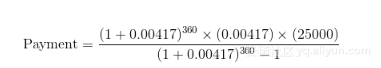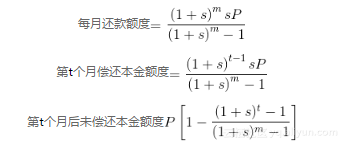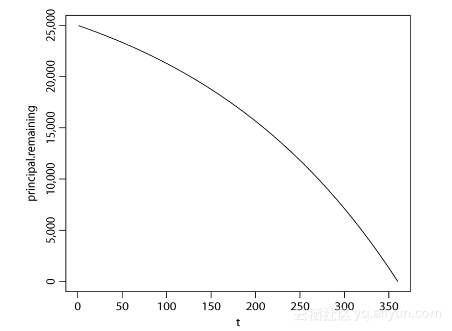#《R语言初学指南》一2.5 实际案例

1. 云栖社区>
2. 博客>
3. 正文

## 《R语言初学指南》一2.5 实际案例

### 2.5 实际案例

R语言初学指南``````> (1+.00417)^360*.00417*25000/((1+.00417)^360-1)
 134.2665
````````````#==============================================
# loan payment.R:　R script to calculate and plot monthly loan
# payment information.
#==============================================
#----------------------------------------------
# Step 0. Assign numerical values to P (principal), m (total
# number of monthly payments), and i (annual interest rate).
# Calculate s (monthly interest rate).
#----------------------------------------------
P=25000
m=360
i=.05　　# Interest is 100*i percent per year.
s=i/12　 # Monthly interest rate.
#----------------------------------------------
# Step 1.　Calculate a vector of values of time (months) going
# from 1 to m.
#----------------------------------------------
t=1:m

#----------------------------------------------
# Step 2.　Calculate the monthly payment (a single number)
# using the first loan equation.
#----------------------------------------------
monthly.payment=(1+s)^m*s*P/((1+s)^m−1)

#----------------------------------------------
# Step 3.　Calculate a vector of principal amounts paid each
# month of the loan using the second loan equation.
#----------------------------------------------
principal.paid.month.t=(1+s)^(t−1)*s*P/((1+s)^m−1)

#----------------------------------------------
# Step 4.　Calculate a vector of principal amounts remaining
# unpaid each month of the loan using the third loan equation.
#----------------------------------------------
principal.remaining=P*(1−((1+s)^t−1)/((1+s)^m−1))

#----------------------------------------------
# Step 5. Calculate a vector of the interest amounts paid
# each month by subtracting the principal amounts paid from the
# monthly payment.
#----------------------------------------------
interest.paid.month.t=monthly.payment-principal.paid.month.t

#----------------------------------------------
# Step 6. Calculate the total interest paid by summing all the
# interest amounts paid each month using the sum( ) function in R.
#----------------------------------------------
total.interest.paid=sum(interest.paid.month.t)

#----------------------------------------------
# Step 7.　Print the results to the console.
#----------------------------------------------
monthly.payment
total.interest.paid
t
principal.paid.month.t
interest.paid.month.t
principal.remaining
#----------------------------------------------
# Step 8. Draw a graph of the principal remaining in the loan
# each month (vertical axis) versus the vector of months
# (horizontal axis).
#----------------------------------------------
plot(t,principal.remaining,type="l")
``````